# How to count the number of characters in a cell

Jan 11, 2020 • edited Jan 12, 2020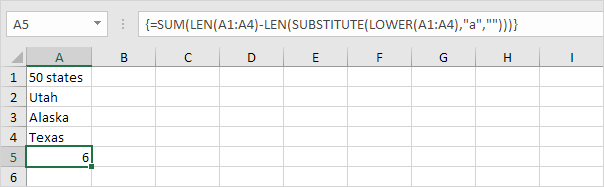When you need to count the characters in cells, use the LEN function—which counts letters, numbers, characters, and all spaces. For example, the length of “It's 98 degrees today, so I'll go swimming” (excluding the quotes) is 42 characters—31 letters, 2 numbers, 8 spaces, a comma, and 2 apostrophes.

To use the function, enter =LEN(cell) in the formula bar, then press Enter on your keyboard.

## Method 1

1. The LEN function in Excel counts the number of characters in a cell.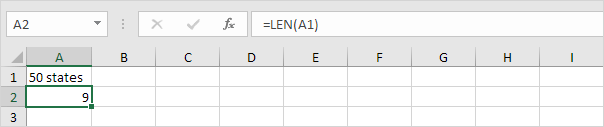Explanation: the LEN function counts 2 numbers, 1 space and 6 letters.

2. You can combine the SUM function and the LEN function to count the number of characters in a range of cells.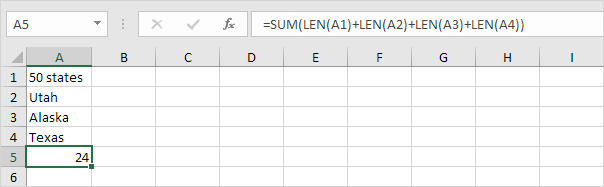Note: as you can imagine, this formula can get quite long.

3. The array formula below counts the number of characters in a range of cells.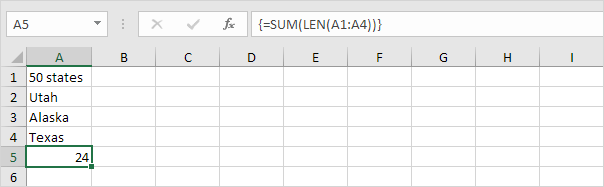Note: finish an array formula by pressing CTRL + SHIFT + ENTER. Excel adds the curly braces {}. The array constant {9;4;6;5} is used as an argument for the SUM function, giving a result of 24. Maybe this is one step too far for you at this stage, but it shows you one of the many other powerful features Excel has to offer.

4. Use the LEN function and the SUBSTITUTE function to count how many times a specific character (in this example, the character a) occurs in a cell.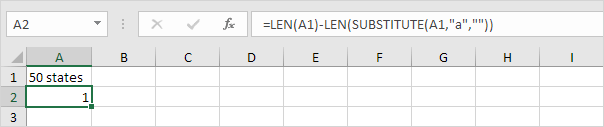Explanation: the SUBSTITUTE function replaces the character a (second argument) with an empty string (third argument). LEN(SUBSTITUTE(A1,“a”,"")) equals 8 (the length of the string without the character a). If we subtract this number from 9 (total number of characters in cell A1), we get the number of occurrences of the character a in cell A1.

5. The array formula below counts how many times a specific character (in this example, the character a) occurs in a range of cells.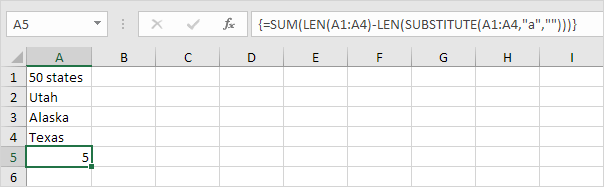Note: finish an array formula by pressing CTRL + SHIFT + ENTER. Excel adds the curly braces {}. The array constant {1;1;2;1} is used as an argument for the SUM function, giving a result of 5. The SUBSTITUTE function is case-sensitive. The A in Alaska is not counted.

6. The array formula below counts both lower and upper case occurrences of a specific character (in this example, the character a).Explanation: The LOWER function converts all letters to lowercase first.

## Method 2

Multiple cells: To apply the same formula to multiple cells, enter the formula in the first cell and then drag the fill handle down (or across) the range of cells.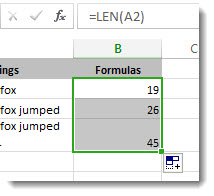To get the a total count of all the characters in several cells is to use the SUM functions along with LEN. In this example, the LEN function counts the characters in each cell and the SUM function adds the counts:

=SUM((LEN( cell1 ),LEN( cell2 ),(LEN( cell3 )) )).

## Give it a try

Here are some examples that demonstrate how to use the LEN function.

Copy the table below and paste it into cell A1 in an Excel worksheet. Drag the formula from B2 to B4 to see the length of the text in all the cells in column A.

Text StringsFormulas
The quick brown fox.=LEN(A2)
The quick brown fox jumped.
The quick brown fox jumped over the lazy dog.

Count characters in one cell

1. Click cell B2.
2. Enter =LEN(A2).

The formula counts the characters in cell A2, which totals to 27—which includes all spaces and the period at the end of the sentence.

NOTE: LEN counts any spaces after the last character.

Count characters in multiple cells

1. Click cell B2.
2. Press Ctrl+C to copy cell B2, then select cells B3 and B4, and then press Ctrl+V to paste its formula into cells B3:B4.

This copies the formula to cells B3 and B4, and the function counts the characters in each cell (20, 27, and 45).

Count a total number of characters

1. In the sample workbook, click cell B6.
2. In the cell, enter =SUM(LEN(A2),LEN(A3),LEN(A4)) and press Enter.

This counts the characters in each of the three cells and totals them (92).

#How To#Tutorial#Functions#Count#characters

What are the most useful shortcuts in Excel

How to copy that cell over to column B without either of those 2 words